# Why Computer Algebra Won’t Cure Your Calculus Blues

Why Computer Algebra Won’t Cure Your Calculus Blues

By Richard Harris

We still haven’t found how to accurately do calculus. Richard Harris revisits an algebraic technique.

We began the second half of this series with a brief history of the differential calculus and a description of perhaps the most powerful mathematical tool for numerical computing, Taylor’s theorem, which states that for a function fwhere f' ( x ) denotes the first derivative of f at x , f" ( x ) denotes the second and f ( n )( x ) the n th and where, by convention, the 0 th derivative is the function itself.

We then used Taylor’s theorem to perform a detailed analysis of finite difference approximations of various orders of derivatives and, in the previous instalment, sought to use polynomial approximations to automate the calculation of their formulae.

We concluded with Ridders’ algorithm [Ridders82] which treated the symmetric finite difference as a function of the change in the argument, δ , and used a polynomial approximation of it to estimate the value of the difference with a δ of zero.

You will no doubt recall that this was a significant improvement over every algorithm we had previously examined with an average error roughly just one decimal order of magnitude worse than the theoretical minimum.

Given that this series of articles has not yet concluded, the obvious question is whether or not we can close that gap.

The obvious answer is that we can.

Sort of.

One of the surprising facts about differentiation is that it is almost always possible to find the expression for the derivative of a function if you have the expression for the function itself. This might not seem surprising until you consider the inverse operation; there are countless examples where having the expression for the derivative doesn’t mean that we can find the expression for the function.

This is enormously suggestive of a method by which we can further improve our calculation of derivatives; get the computer to generate the correct expression for us.

## Computer algebra revisited

We first discussed computer algebra as part of our quest to find an infinite precision numeric type [Harris11]. The idea was to represent an expression as a tree rather than directly compute its value, as shown in figure 1 (an expression tree for the golden ratio).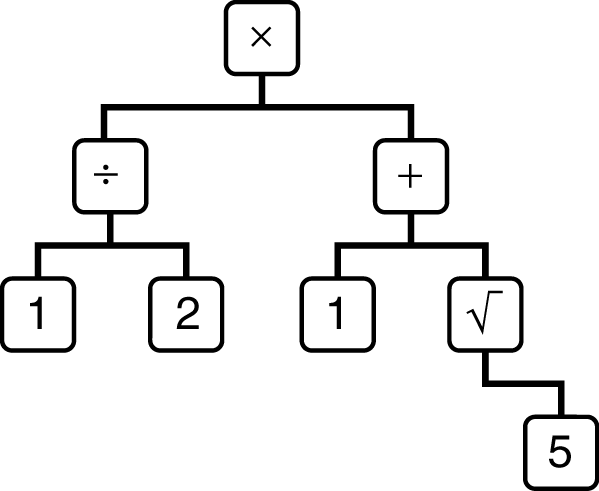Figure 1

A common implementation of such expression trees uses a pure virtual base class to represent the nodes. Our original attempt is given in listings 1 (the original expression object base class) and 2 (the original expression wrapper class).

 ```class expression_object { public: enum{empty=0xE}; virtual ~expression_object() {}; virtual double approx() const = 0; virtual unsigned char exact(const bignum &n) const = 0; virtual bignum::sign_type sign() const = 0; }; ``` Listing 1
 ```class expression { public: typedef shared_ptr object_type; enum{empty=expression_object::empty}; expression(); expression(const bignum &x); explicit expression(const object_type &x); double approx() const; unsigned char exact(const bignum &n) const; bignum::sign_type sign() const; object_type object() const; int compare(const expression &x) const; expression & negate(); expression & operator+=(const expression &x); expression & operator-=(const expression &x); expression & operator*=(const expression &x); expression & operator/=(const expression &x); private: object_type object_; }; ``` Listing 2

You may recall that my proposal to compute the digits of the decimal expansion of the expression one at a time with the ``` exact ``` member function (with negative indices to the left of the decimal point and positive to the right) as a means of effectively maintaining infinite precision was ultimately doomed to failure because of the impossibility of comparing equal values. There was simply no way, in general, to decide when to give up.

However, the simplicity and near universal applicability of the rules of differentiation gives these expression objects something of a reprieve; they are supremely well suited for automating those rules.

## Symbolic differentiation

We shall, of course, need to redesign our expression object classes; they were after all rather useless in their original form.

We shall therefore do away with the notion of exact evaluation and content ourselves with just floating point and shall further add a virtual member function that returns the expression representing the derivative, as shown in listings 3 (the new expression object base class) and 4 (the new expression wrapper class).

 ```class expression_object { public: virtual ~expression_object() {}; virtual double value() const = 0; virtual expression derivative(const expression &x) const = 0; }; ``` Listing 3
 ```class expression { public: typedef shared_ptr object_type; expression(); expression(double x); explicit expression(const object_type &x); double value() const; expression derivative(const expression &x) const; object_type object() const; expression & negate(); expression & operator+=(const expression &x); expression & operator-=(const expression &x); expression & operator*=(const expression &x); expression & operator/=(const expression &x); private: object_type object_; }; ``` Listing 4

Note that the ``` value ``` member function replaces the ``` approximate ``` member function and will have identical implementations in derived classes, as illustrated in listings 5 (the new substraction expression class) and 6 (the ``` value ``` member function of ``` subtraction_expression ``` ).

 ```class subtraction_expression : public expression_object { public: explicit subtraction_expression( const expression &lhs, const expression &rhs); virtual ~subtraction_expression(); virtual double value() const; virtual expression derivative(const expression &x) const; const expression lhs; const expression rhs; }; ``` Listing 5
 ```double subtraction_expression::value() const { return lhs.value() - rhs.value(); } ``` Listing 6

There’s little to be gained in repeating this simple change for all types of expression so we shall take it as read that we have defined a full suite of expression objects and implemented their value member functions so that we can concentrate on the implementation of the ``` derivative ``` member functions.

The ``` expression ``` wrapper class simply forwards the call to ``` derivative ``` to the underlying ``` expression_object ``` as shown in listing 7.

 ```expression expression::derivative(const expression &x) const { return object_->derivative(x); } ``` Listing 7

The first of the underlying expression objects we shall consider is the ``` constant_expression ``` , which should trivially return 0 in all cases, as shown in listing 8.

 ```expression constant_expression::derivative (const expression &x) const { return expression(0.0); } ``` Listing 8

Next up is the ``` variable_expression ``` which shall serve as the type we differentiate by. As such it should return 1 if differentiated by itself and 0 otherwise, as illustrated in listing 9.

 ```expression variable_expression::derivative (const expression &x) const { return expression (x.object().get()==this ? 1.0 : 0.0); } ``` Listing 9

Addition and subtraction expression object are barely less trivial to differentiate than constants and variables, as illustrated in listing 10.

 ```expression addition_expression::derivative (const expression &x) const { return lhs.derivative(x) + rhs.derivative(x); } expression subtraction_expression::derivative (const expression &x) const { return lhs.derivative(x) - rhs.derivative(x); } ``` Listing 10

Now that we’ve got these trivial cases out of the way we’re ready to implement some of the more subtle rules of differentiation. We shall start with the product rule which states thatThis identity is reflected in the implementation of the ``` derivative ``` member function of the ``` multiplication_expression ``` class given in listing 11.

 ```expression multiplication_expression::derivative (const expression &x) const { return lhs * rhs.derivative(x) + rhs * lhs.derivative(x); } ``` Listing 11

Division is slightly more complicated since it relies upon the chain rule which states thatIn the case of division we are effectively multiplying one function by the reciprocal of another, so we must apply both the chain rule and the product rule.An implementation is given in listing 12.

 ```expression division_expression::derivative (const expression &x) const { const expression &dl = lhs.derivative(x); const expression &dr = rhs.derivative(x); return -dr*lhs/(rhs*rhs) + dl/rhs; } ``` Listing 12

The chain rule is absolutely fundamental in working out how to implement the ``` derivative ``` member function for all of the remaining expression objects.

As a final example, we shall take a look at raising one expression to the power of another. Now it is not entirely obvious how to do this. Differentiating a variable raised to a constant power should be familiar enoughHowever, differentiating a constant raised to the power of a variable isn’t quite so straightforwardThe trick is to exponentiate the logarithm of c xafter which the result is trivially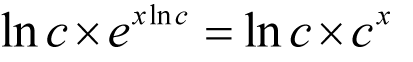Combining this with the chain rule, the product rule and the fact that the derivative of the exponential is equal to itself yieldsListing 13 illustrates an implementation of the derivative of a power expression.

 ```expression power_expression::derivative (const expression &x) const { return (log(lhs)*rhs.derivative(x) +rhs*lhs.derivative(x)/lhs) * expression(expression::object_type(this)); } ``` Listing 13

I shall leave the implementation of further expression objects to you and move on to consider the implications of this approach.

## Exact differentiation?

On the face of it we have finally achieved what we set out to do; implement an algorithm that can calculate the derivative of a function to machine precision.

In using expression objects to symbolically differentiate expressions we are able to generate an expression that is mathematically identical to the derivative.

All that remains is to evaluate it.

Unfortunately there are a few problems.

## Memory use

The first problem is that the expressions representing derivatives can grow in complexity at an alarming rate.

For example, consider the functionDifferentiating with respect to x yieldsDifferentiating again yieldsOnce more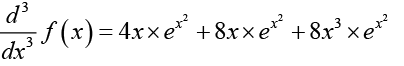Clearly the trees representing these expressions will grow quite rapidly unless we algebraically simplify them. For example, the third derivative can be simplified toreducing the number of arithmetic operations from 15 to 7.

Unfortunately implementing a computer algebra system that is capable of performing such simplifications is no easy task. Much like chess programs they require large databases of valid transformations of expressions, some heuristic that accurately captures our sense of simplicity and expensive brute-force search algorithms to traverse the variety of ways in which those transformations can be applied.

Unless we are willing to expend a very great deal more effort we shall have to accept the fact that symbolic differentiation will be something of a memory hog.

## Cancellation error

The next problem also stems from the difficulty in simplifying expressions but is rather more worrying. Consider redundant expression which though complex, when fully simplified, yield trivial expressions.

As an example considerTrivially, this has a derivative of 0 for all x , but when we apply our expression objects to compute the derivative, they will blindly follow the rules to yieldMathematically, this is identically equal to 0 for all x , but since we are using floating point some errors will inevitably creep in. We shall consequently be subtracting two nearly equal numbers which is the very incantation we use to summon the dreaded cancellation error.

Figure 2 illustrates the result of this calculation for x from 0.01 to 1 in steps of 0.01 and clearly shows the effect of cancellation on the result.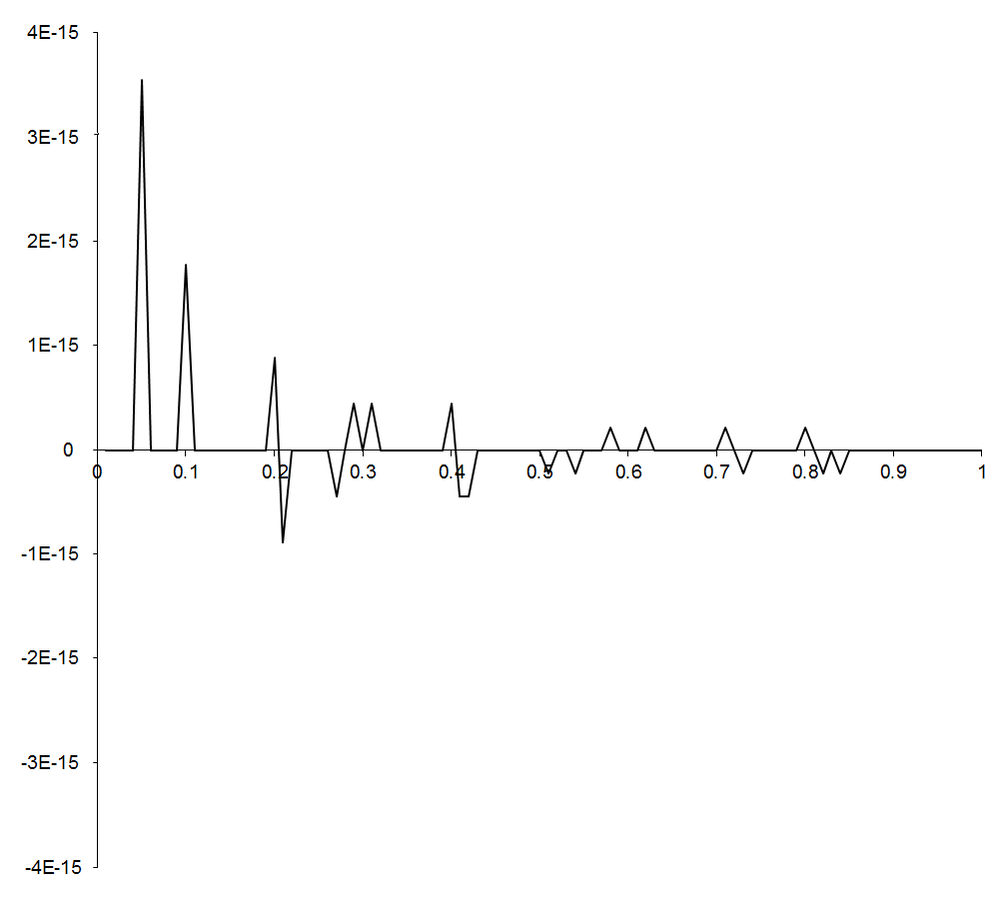Figure 2

Whilst this is a simple example, it is indicative of a wider problem. Even if we have taken great care to ensure that the expression representing a function is not susceptible to cancellation error we do not know whether the same can be said of the expression representing its derivative.

We can at least use interval arithmetic to monitor numerical error in the calculation of derivatives. You will recall that interval arithmetic works by placing bounds on arithmetic operations by computing the worst case smallest and largest results given the bounds on its arguments and numerical rounding.

The simple change to the expression object base class is illustrated in listing 14.

 ```class expression_object { public: virtual ~expression_object() {} virtual interval value() const = 0; virtual expression derivative(const expression &x) const = 0; }; ``` Listing 14

Figure 3 shows the result of evaluating the expression for the derivative of x / x using interval arithmetic and clearly reveals the presence of significant cancellation error near 0.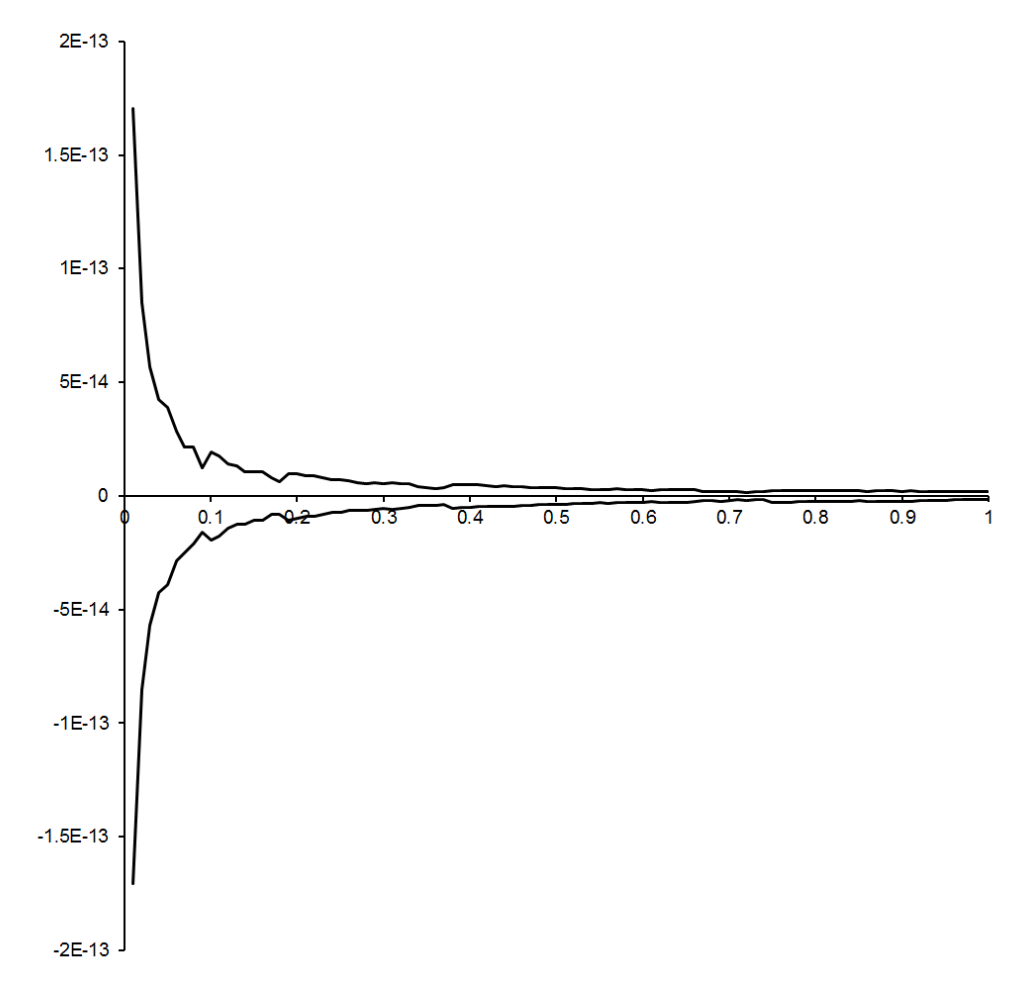Figure 3

This combination of expression objects and interval arithmetic is as effective an algorithm for the calculation of derivatives as is possible without implementing a fully functional computer algebra system.

In practice, the principal drawback is in the expense of maintaining the expression tree and requiring virtual function calls to evaluate even simple arithmetic operations, although admittedly the latter can be largely avoided with the judicious use of templates.

## Numerical approximation

There are many mathematical functions for which we have no simple formulae and must instead rely upon approximations. These approximations are often iterative in nature, stopping when some convergence criteria is satisfied.

If we naively implement an iterative approximation using an expression object as the argument we shall almost certainly run into trouble.

Not only is the resulting expression tree liable to be extremely large, it may take a different form for different values of the argument. If we generate an approximating expression using the initial value of the argument, we may very well introduce significant errors for other values.

To accurately represent iterative functions with expression objects we shall need to implement expression objects representing loops, conditional statements and so on and so forth.

Unfortunately working out the symbolic derivatives of such expressions is, in general, a tricky proposition.

Assuming that we have done so, or that the approximation takes the same form for all values of the argument, we still aren’t quite out of the woods. The derivative of an accurate approximating expression for a function is not necessarily an accurate approximating expression for the derivative of that function.

For example, consider the functionwhich serves as an approximation to f ( x ).

Now, this is trivially everywhere equal to within 1 percent of the value of f ( x ), but if we calculate its derivative we find thatwhich can differ from the actual derivative by as much as 100 percent of the value of f ( x )!

In such cases we may be better served by a polynomial approximation algorithm since these can effectively smooth out this high frequency noise by terminating once the derivative starts growing as δ shrinks close to its wavelength.

This observation hints at how we must proceed if we wish to use expression objects in conjunction with numerical approximations of functions.

Rather than represent the approximation of the function with an expression tree, we must create a new type of expression object that implements the approximation. The ``` derivative ``` member function must then return an expression object representing the derivative of the function.

In the worst case this may simply be an expression object implementing a polynomial approximation algorithm. Better still would be an expression object implementing a specifically designed approximation of the derivative. Best of all is the case when the derivative is known in closed form, such as the derivative of a numerical integration for example, in which case it can be an expression tree.

If necessary we can further implement an entire family of expression objects representing approximations of higher order derivatives to allow for higher orders of differentiation.

For these reasons I declare this approach to be a standing army of ducks; an effective fighting force, but rather expensive to feed and not always entirely welcome.

Quack two three four! Quack two three four!

[Harris11] Harris, R., Overload , Issue 102, ACCU, 2011.

[Ridders82] Ridders, C.J.F., Advances in Engineering Software , Volume 4, Number 2., Elsevier, 1982.